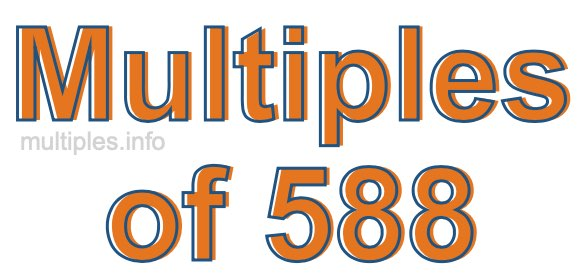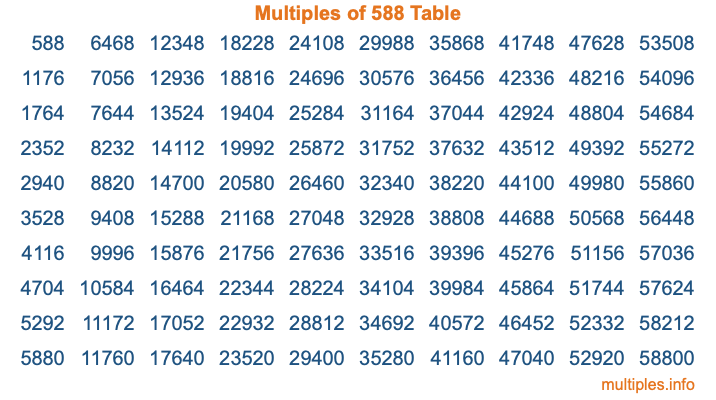Multiples of 588Welcome to the Multiples of 588 page. Here we will first teach you everything you will ever need to know about the multiples of 588, and then give you a study guide summary of everything we taught you to make sure you remember it all. Use this page to look up facts and learn information about the multiples of 588. This page will make you a multiples of five hundred eighty-eight expert!

Definition of Multiples of 588
Multiples of 588 are all the numbers that when divided by 588 equal an integer. Each of the multiples of 588 are called a multiple. A multiple of 588 is created by multiplying 588 by an integer.

Therefore, to create a list of multiples of 588, you start with 1 multiplied by 588, then 2 multiplied by 588, then 3 multiplied by 588, and so on for as long as you want. Thus, the list of the first five multiples of 588 is 588, 1176, 1764, 2352, and 2940. To see a larger list of multiples of 588, see the printable image of Multiples of 588 further down on this page. We also have a category where you can choose any nth multiple of 588.

Multiples of 588 Checker
The Multiples of 588 Checker below checks to see if any number of your choice is a multiple of 588. In other words, it checks to see if there is any number (integer) that when multiplied by 588 will equal your number. To do that, we divide your number by 588. If the the quotient is an integer, then your number is a multiple of 588.

Is  a multiple of 588?

Least Common Multiple of 588 and ...
A Least Common Multiple (LCM) is the lowest multiple that two or more numbers have in common. This is also called the smallest common multiple or lowest common multiple and is useful to know when you are adding our subtracting fractions. Enter one or more numbers below (588 is already entered) to find the LCM.

Check out our LCM Calculator if you need more details about the Least Common Multiple or if you need the LCM for different numbers for adding and subtraction fractions.

nth Multiple of 588
As we stated above, 588 is the first multiple of 588, 1176 is the second multiple of 588, 1764 is the third multiple of 588, and so on. Enter a number below to find the nth multiple of 588.

th multiple of 588

Multiples of 588 vs Factors of 588
588 is a multiple of 588 and a factor of 588, but that is where the similarities end. All postive multiples of 588 are 588 or greater than 588. All positive factors of 588 are 588 or less than 588.

Below is the beginning list of multiples of 588 and the factors of 588 so you can compare:

Multiples of 588: 588, 1176, 1764, 2352, 2940, etc.

Factors of 588: 1, 2, 3, 4, 6, 7, 12, 14, 21, 28, 42, 49, 84, 98, 147, 196, 294, 588

As you can see, the multiples of 588 are all the numbers that you can divide by 588 to get a whole number. The factors of 588, on the other hand, are all the whole numbers that you can multiply by another whole number to get 588.

It's also interesting to note that if a number (x) is a factor of 588, then 588 will also be a multiple of that number (x).

Multiples of 588 vs Divisors of 588
The divisors of 588 are all the integers that 588 can be divided by evenly. Below is a list of the divisors of 588.

Divisors of 588: 1, 2, 3, 4, 6, 7, 12, 14, 21, 28, 42, 49, 84, 98, 147, 196, 294, 588

The interesting thing to note here is that if you take any multiple of 588 and divide it by a divisor of 588, you will see that the quotient is an integer.

Multiples of 588 Table
Below is an image of the first 100 multiples of 588 in a table. The table is in chronological order, column by column. The first column has the first ten multiples of 588, the second column has the next ten multiples of 588, and so on.The Multiples of 588 Table is also referred to as the 588 Times Table or Times Table of 588. You are welcome to print out our table for your studies.

Negative Multiples of 588
Although not often discussed or needed in math, it is worth mentioning that you can make a list of negative multiples of 588 by multiplying 588 by -1, then by -2, then by -3, and so on, to get the following list of negative multiples of 588:

-588, -1176, -1764, -2352, -2940, etc.

Multiples of 588 Summary
Below is a summary of important Multiples of 588 facts that we have discussed on this page. To retain the knowledge on this page, we recommend that you read through the summary and explain to yourself or a study partner why they hold true.

There are an infinite number of multiples of 588.

A multiple of 588 divided by 588 will equal a whole number.

588 divided by a factor of 588 equals a divisor of 588.

The nth multiple of 588 is n times 588.

The largest factor of 588 is equal to the first positive multiple of 588.

588 is a multiple of every factor of 588.

588 is a multiple of 588.

A multiple of 588 divided by a divisor of 588 equals an integer.

588 divided by a divisor of 588 equals a factor of 588.

Any integer times 588 will equal a multiple of 588.

Multiples of a Number
Here you can get the multiples of another number, all with the same attention to detail as we did for multiples of 588 on this page.

Multiples of
Multiples of 589
Did you find our page about multiples of five hundred eighty-eight educational? Do you want more knowledge? Check out the multiples of the next number on our list!